# Module 4: Sowing A Crop

Created 23/02/2023

IMPORTANT NOTE: It is highly recommended that you upgrade your APSIM Next Gen version to at least version 2023.2.7164.0 or later.

## Sowing A Crop

In this exercise you will observe the growth of a crop over a single season. You will learn a bit more about how to use APSIM to do a ‘what-if’ experiment with fertiliser rates. These skills can not only be used to experiment with sowing fertiliser rates but also variables such as:

• Time of planting.
• Rate of sowing.
• Crop comparisons and different starting soil moisture conditions.
1. Start a new simulation using the `Wheat.apsimx` example.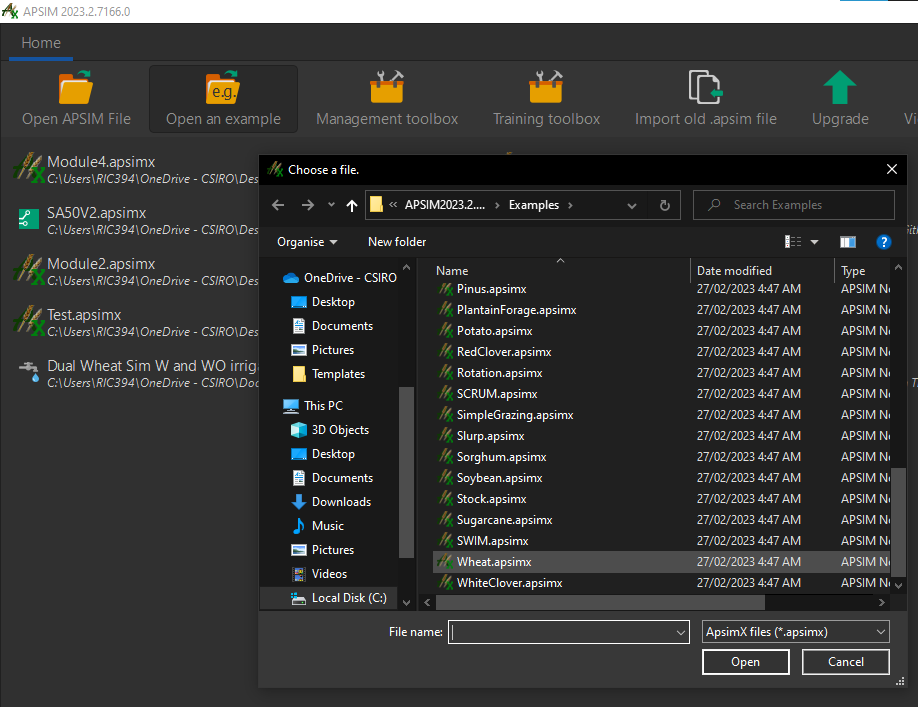• you can find this in the `open an example` menu item
2. Rename the simulation as `Wheat`.
3. Save this simulation as `Module4`.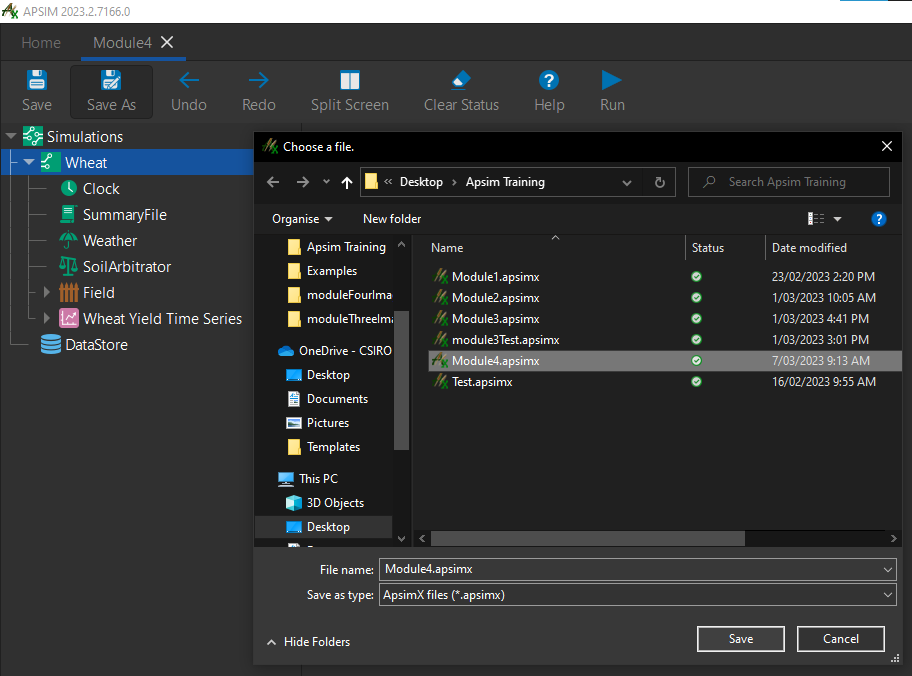4. Make sure that `Dalby.met` is the selected met file under the weather node.
5. Set the start and end dates of the simulation as `1/01/1989 - 31/12/1989` under the `Clock` node.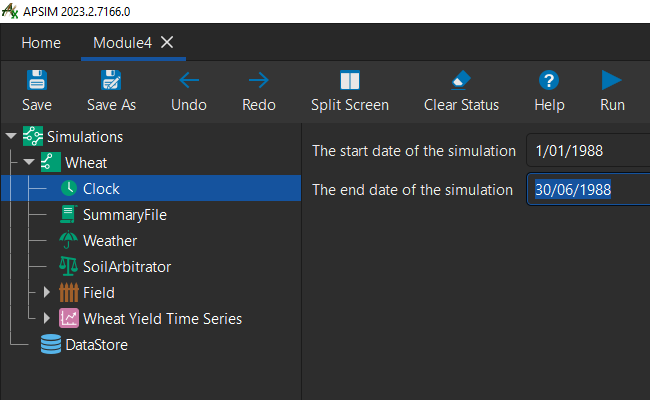6. Set the starting water to 25% full - filled from top. This is under `Soil` then `Water`.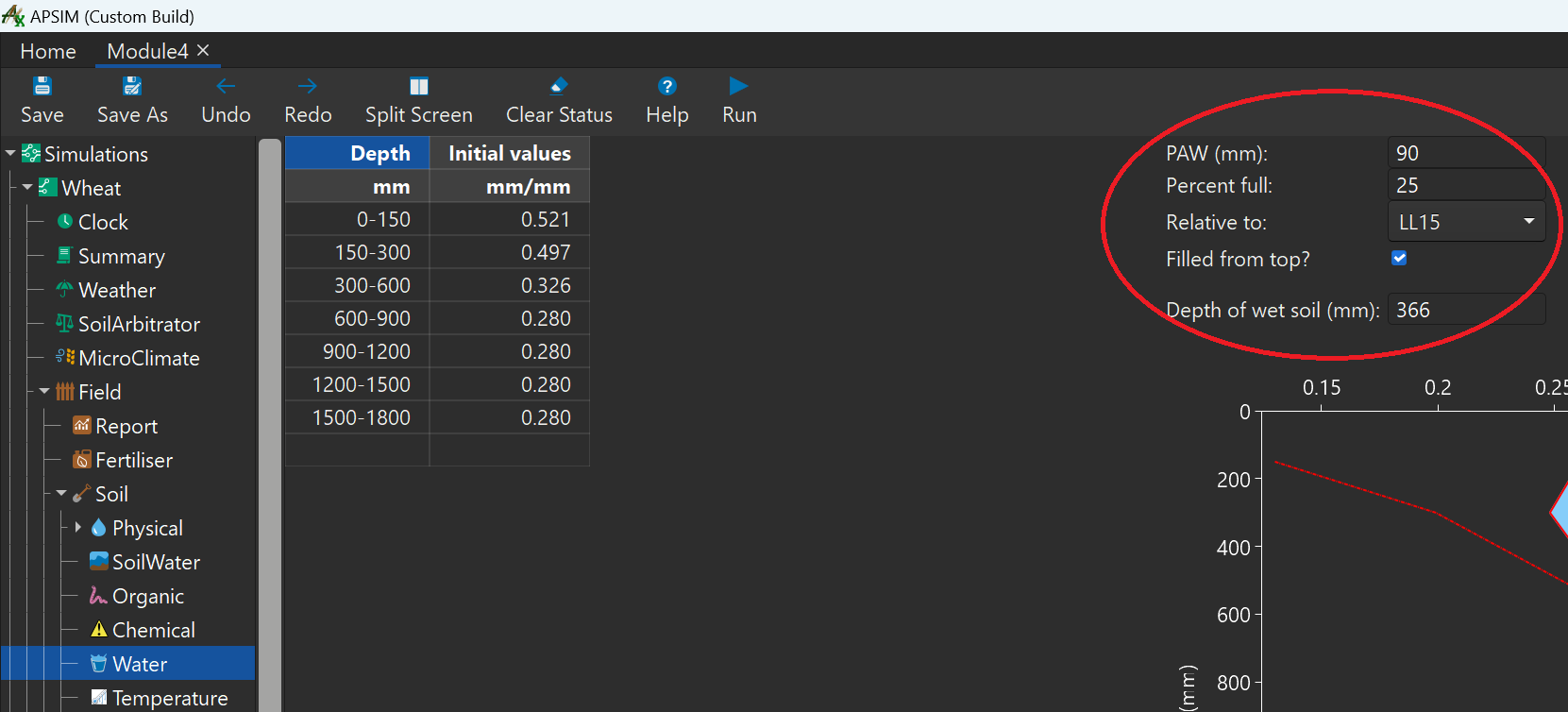7. Set the `initial values` column of both `NO3` and `NH4` to `kg/ha`.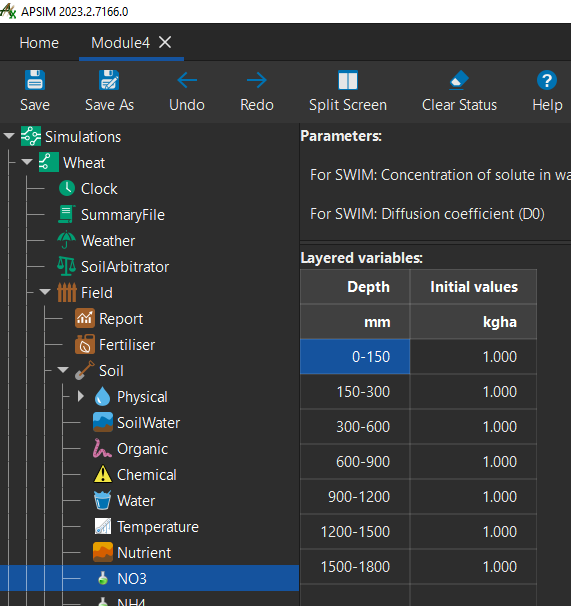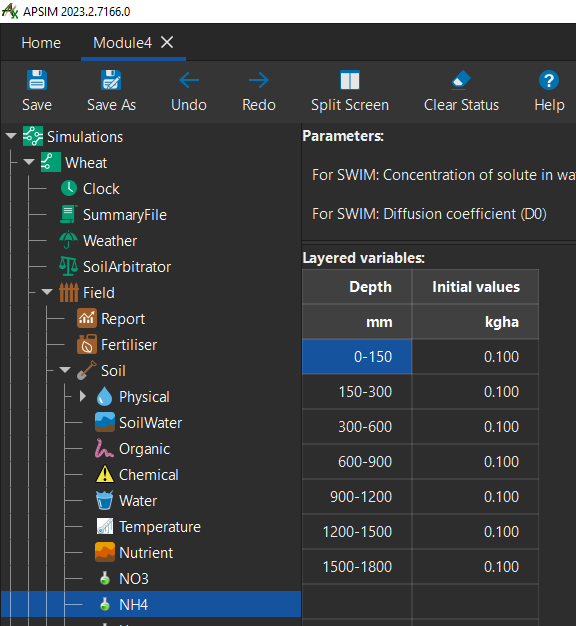8. Now let’s make some changes to the `SowingRule` management node.
9. Change the `SowingFertiliser` parameter `Amount of fertiliser to be applied (kg/ha)` to `0`: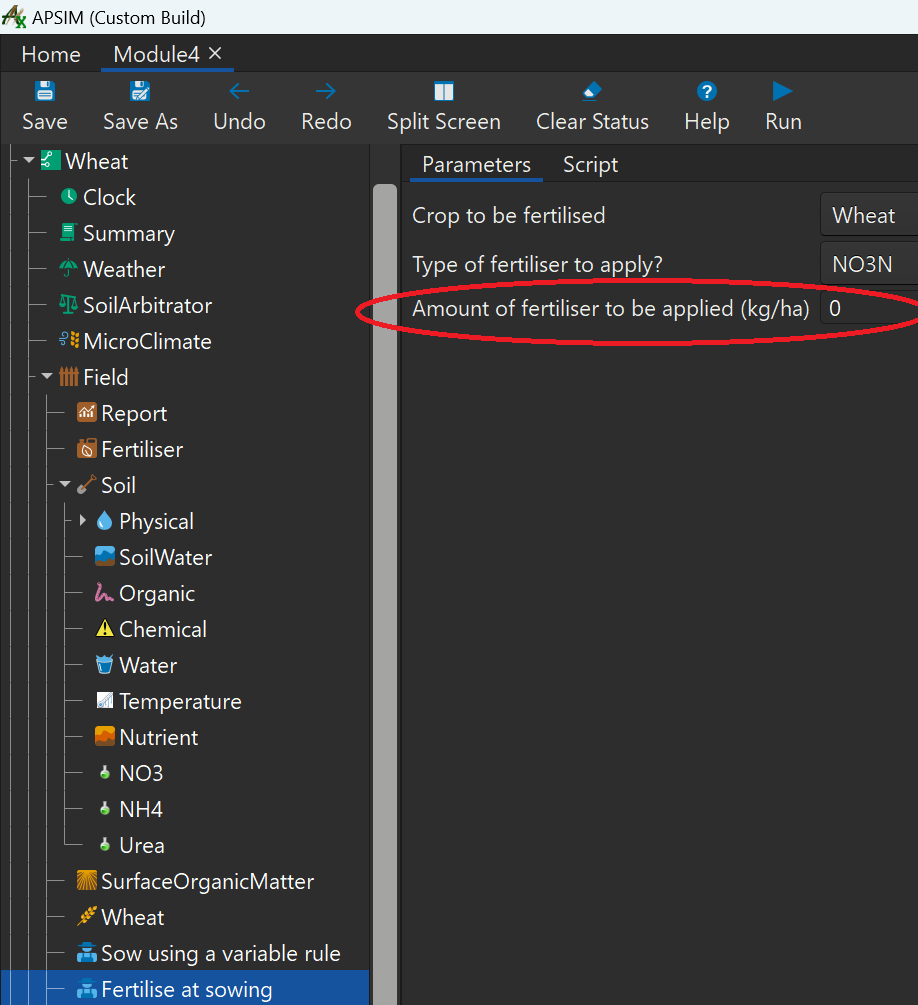10. Let’s run the simulation and then inspect the `Wheat yield time series` graph.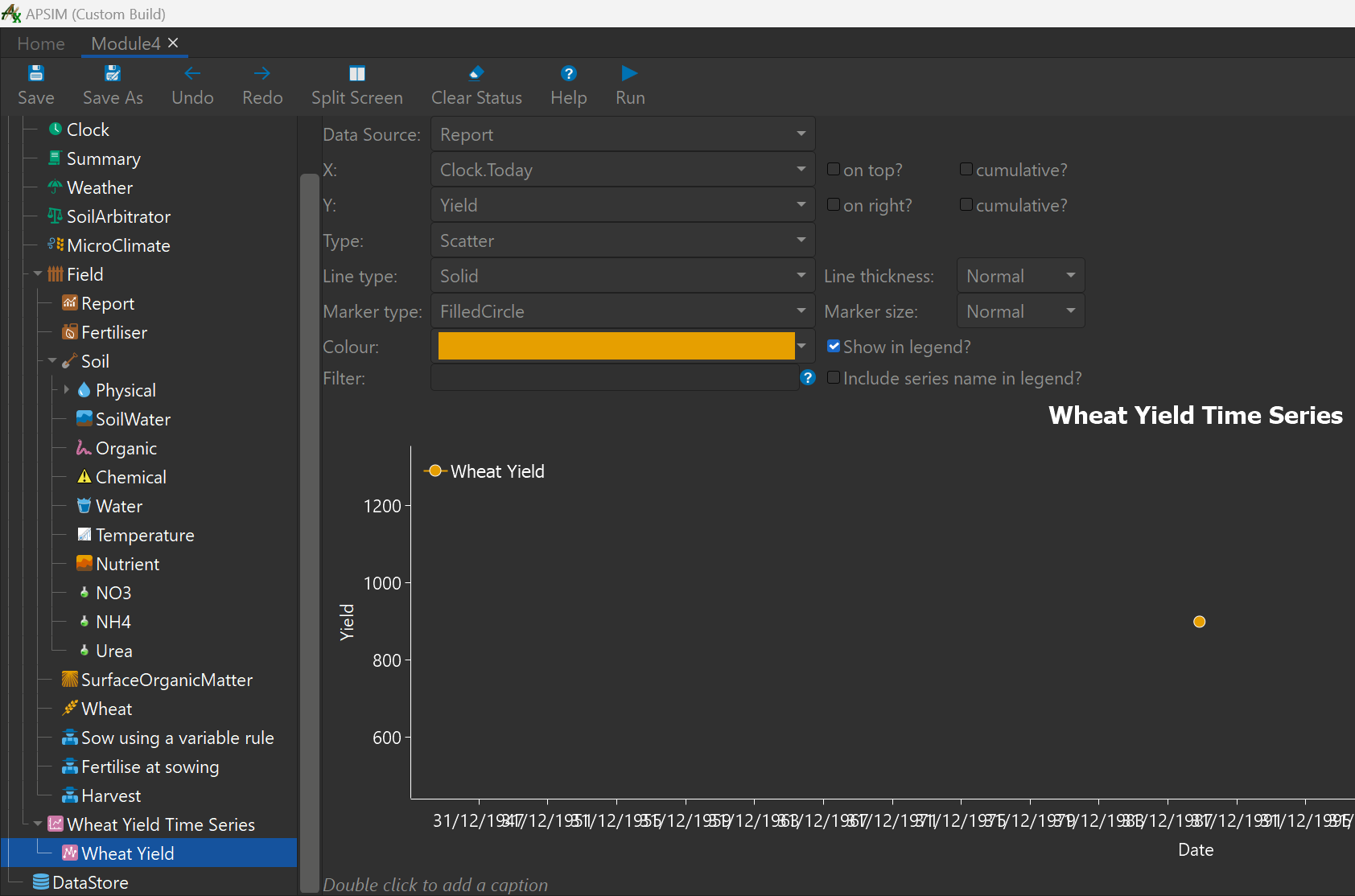11. We can see with 0 sowing fertiliser we achieved a yield of almost 800 kg/ha.
12. Next we will create an experiment where we alter the sowing fertiliser amount for this year. We will see how this affects the yield.

## Creating an experiment

1. First add a `Experiment` node to the `Simulations` node at the top of the simulations tree.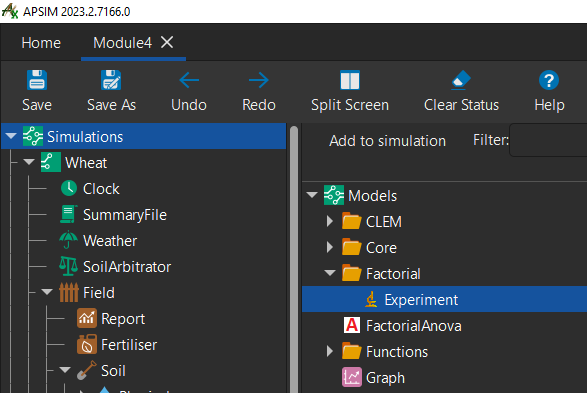2. The `Experiment` node will be added to the bottom of the tree, hold `ctrl` key and press `up arrow` several times until it is directly below the `Simulations` node.
3. Add a `Factors` node to this experiment.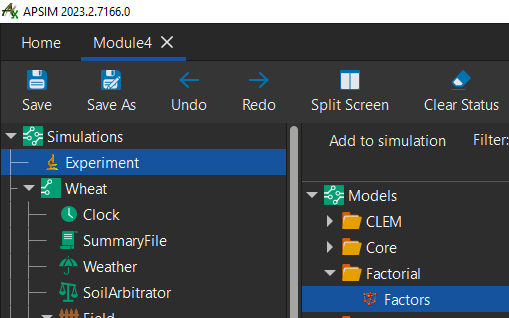4. Add a `Factor` node to the `Factors` node.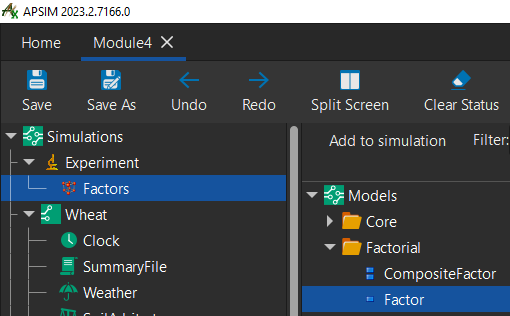5. To be able to change factors in our experiment, we will have to add our `Wheat` simulation to the experiment as a child node.
6. Drag and drop the `Wheat` simulation node onto the `Experiment` node.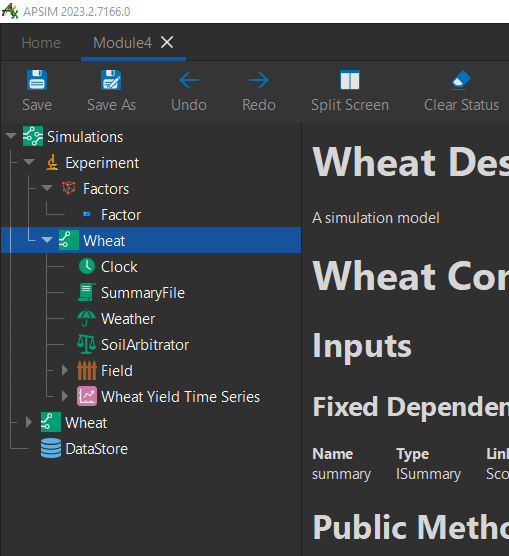7. Delete the `Wheat` simulation that is not a child of the experiment node.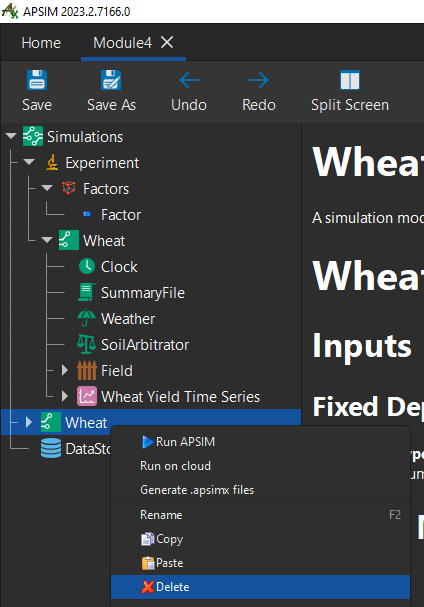8. Next, we will create several versions of our experiment with varying sowing fertiliser amounts.
9. To do this, add this line to the `Factor` node: `[SowingFertiliser].Script.Amount = 0 to 60 step 30`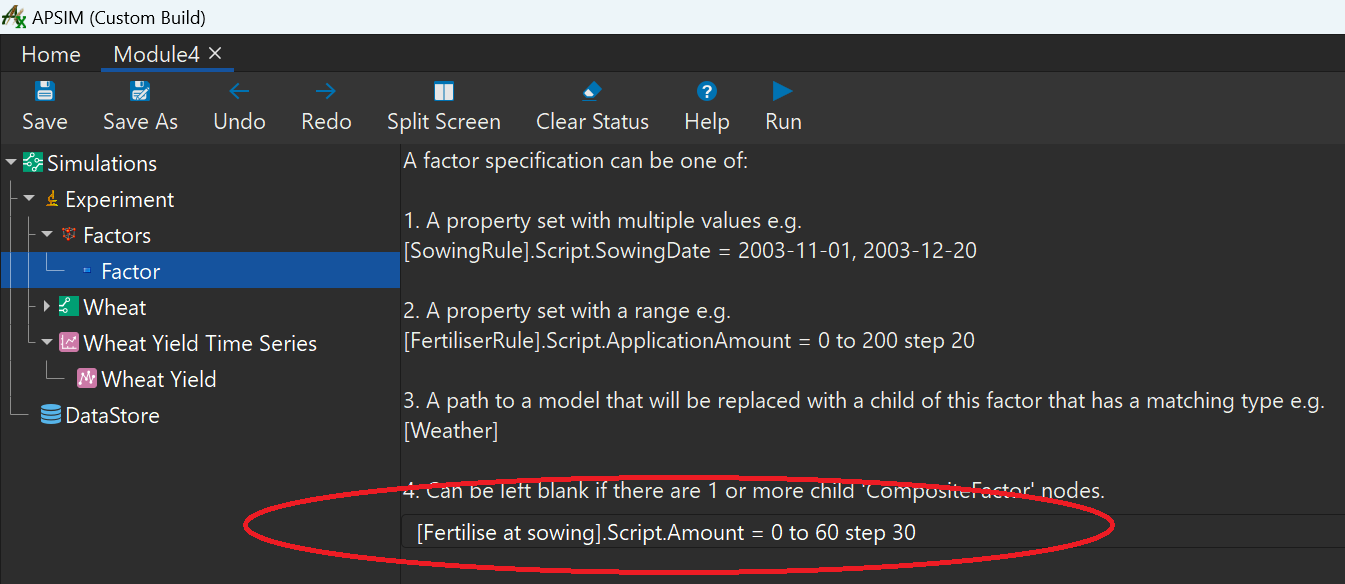10. If we click back on the `Experiment` node, you can see 3 differing amounts of sowing fertiliser.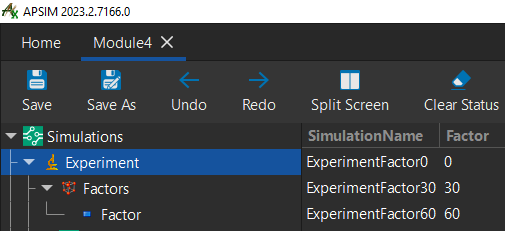11. To see the results lets drag a copy of the `Wheat Yield Time Series` graph onto the `Experiment` node. Change the variables to reflect the image below: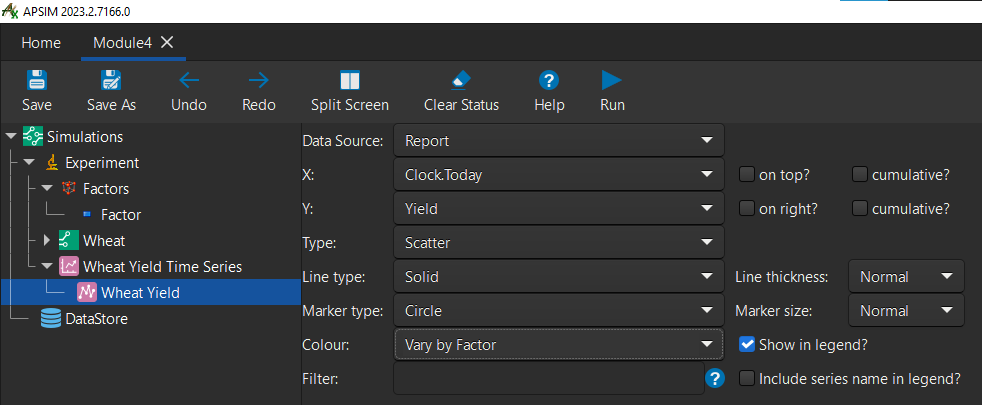12. Run the simulation. You’ll see that the sowing fertiliser amounts increase the yield of the wheat crop by varying degrees.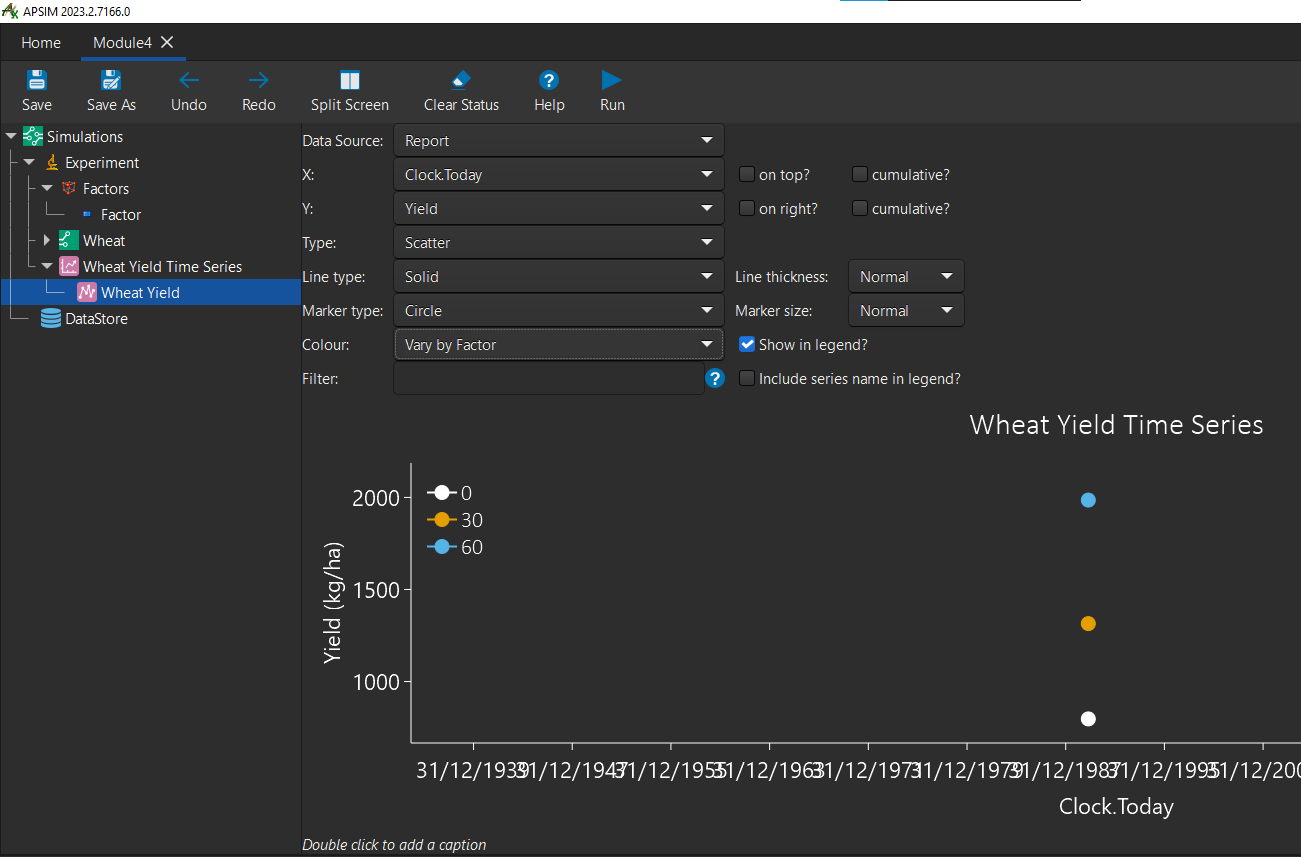Congratulations on completing the 4th module